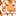Announcements
You can succeed best and quickest by helping others to succeed. Join the conversation.
cancel
Showing results for
Did you mean:Contributor

## Controlling Time Periods shown using a variable in line chart

Hi,

I am trying to create a line chart showing revenue by qtr..  I want to control the quarters shown using a variable.

For example, if I select 4, the line chart should limit to the latest four quarters, if 8 then latest 8 quarters and so on.

I used limiting dimension using fixed number using the variable I created. But, it calculates using the measure I am using  (revenue). This is not the result I want. The variable should only affect the x axis dimension - qtr. in my case.

Please see the sample qvf attached.

Any suggestions are welcome.

Thanks,

Abhishek.

1 Solution

Accepted SolutionsContributor
Author

Hi Guys,

So found a temp solution:

What we need is a calculated dimension. So we need to find a way to rank Qtrs. in decreasing order and then use a calculated dimension for x axis.

=if(Rank<= \$(vQuarters), Qtrs).

Here, the rank variable ranks the latest qtr as 1. For example, if 2021 Q4 is the latest qtr, its rank is 1, 2021 Q3's ranks is 2 and so on.

Though, I feel  this is a workaround. Any better suggestions are welcome.

Thanks,

Abhishek.Contributor
Author

Hi Guys,

So found a temp solution:

What we need is a calculated dimension. So we need to find a way to rank Qtrs. in decreasing order and then use a calculated dimension for x axis.

=if(Rank<= \$(vQuarters), Qtrs).

Here, the rank variable ranks the latest qtr as 1. For example, if 2021 Q4 is the latest qtr, its rank is 1, 2021 Q3's ranks is 2 and so on.

Though, I feel  this is a workaround. Any better suggestions are welcome.

Thanks,

Abhishek.Tags
Community Browser